# OASP笔记 - 16

## 化学丰度分析

Posted by mingjie on November 27, 2018

## 生长曲线法

### 一些计算

$F_\nu = 2\pi \int_{-\infty}^{\infty} B_\nu(\tau_0) E_2(\tau_\nu) \frac{[l_\nu(\tau_0)+\kappa_\nu(\tau_0)]\tau_0 d\log{\tau_0}}{\kappa_0(\tau_0)\log{e}}$

$W = \int_0^\infty \frac{F_\nu-F_c}{F_c}d\nu$

### Scaling relations

$\frac{F_c-F_\nu}{F_c} \approx \text{constant} \frac{l_\nu}{\kappa_\nu} \tag{16.1}$

$w = \frac{\text{constant}}{\kappa_\nu} \int_0^\infty l_\nu d\nu$

$w = \text{constant}\frac{\pi e^2}{mc} \frac{\lambda^2}{c} f \frac{N}{\kappa_\nu} \tag{16.2}$

$$\rho$$被丢到了常数里面。引入元素丰度$$A = N_E/N_H$$以及某元素第$$j$$级电离的原子数$$N_j$$，式子$$(1.17)$$变为：

$N = A \frac{N_j}{N_E} N_H \frac{g_n}{u(T)} e^{\frac{-\chi}{kT}} \tag{16.3}$

$\log{\frac{w}{\lambda}} = \log{C} + \log{A} + \log{g_nf\lambda} - \theta_{ex} \chi - \log{\kappa_\nu} \tag{16.4}$

(MOOG也用了这条式子)$$\frac{w}{\lambda}$$是为了将和多普勒有关的现象归一化（介又是什么？）。上式说明了右侧后面这四项的地位是一致的，所以当其他三个固定的时候，剩下那个就可以作为横坐标出现在生长曲线图上了。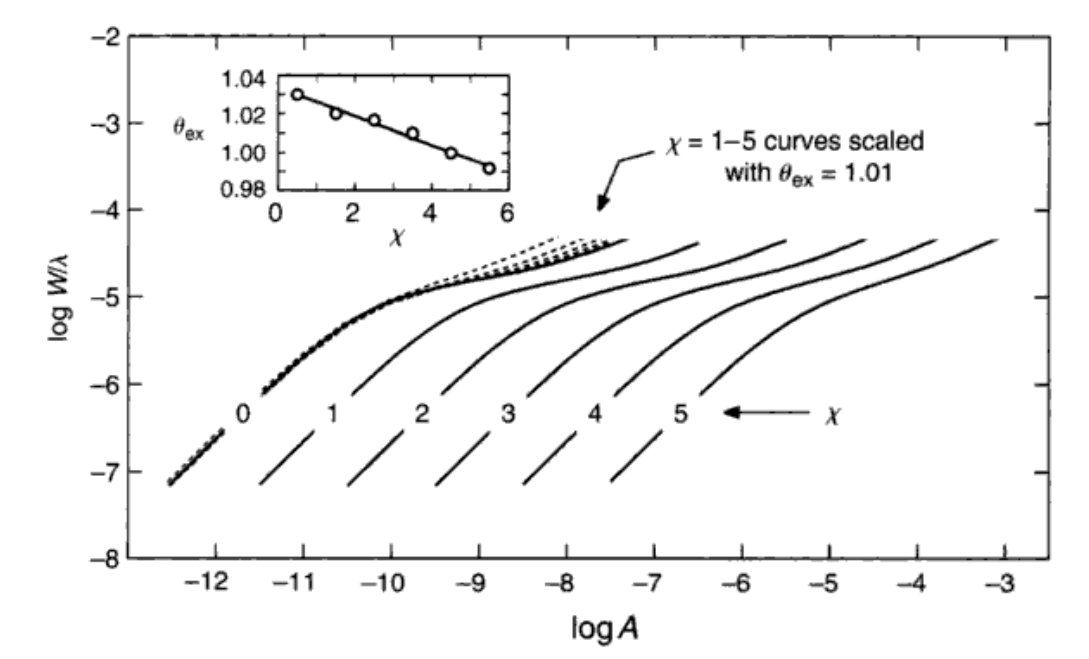### 温度的影响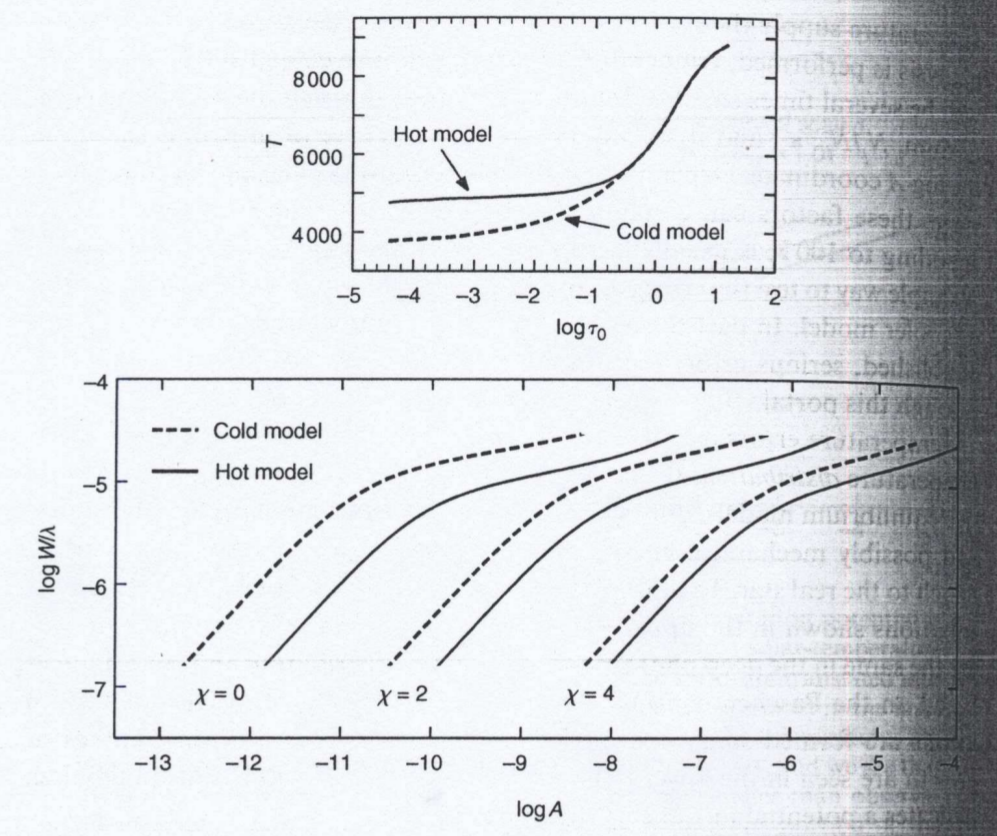### $$\log{g}$$的影响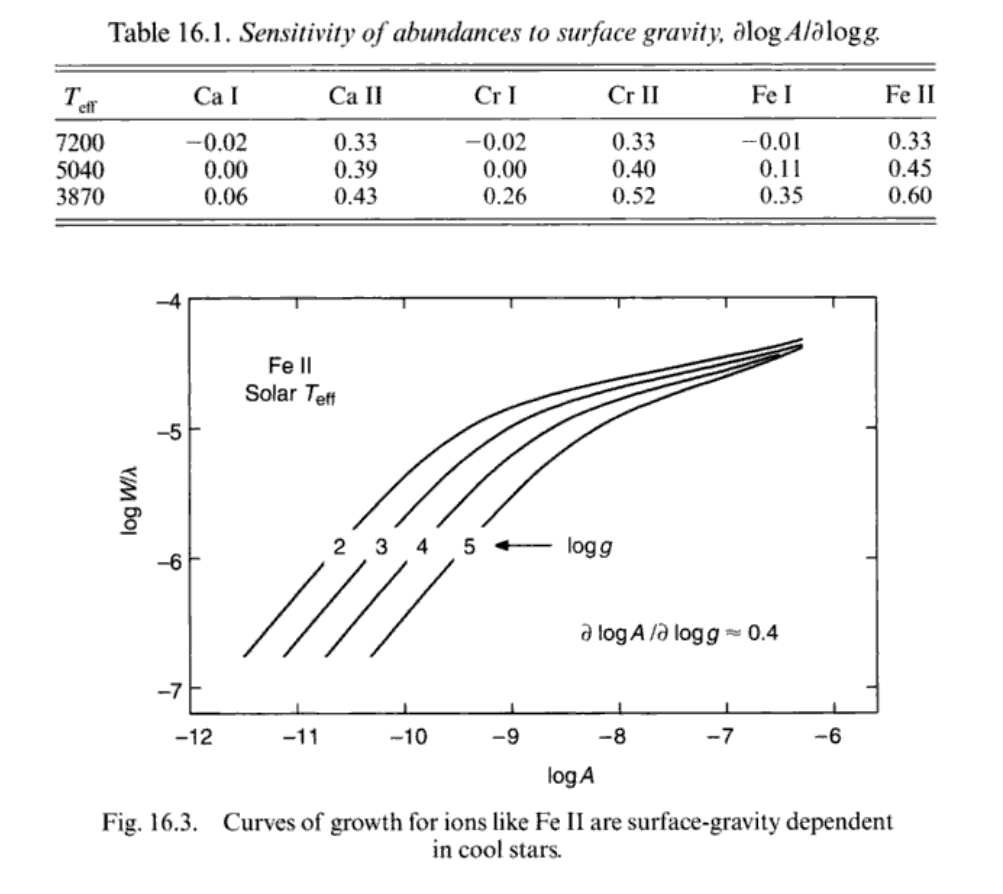### 饱和部分

microturbulence($$\xi$$)，$$\xi$$会将生长曲线的饱和部分拉高，并延迟饱和的点。当然这个特性也可以用来确定$$\xi$$的值，只要先用线性部分确定基本参数、再用不同$$\xi$$来拟合生长曲线的饱和部分就行了。超精细分裂以及磁场分裂造成的影响和$$\xi$$差不多，不过超精细的影响对不同种类的原子不一样。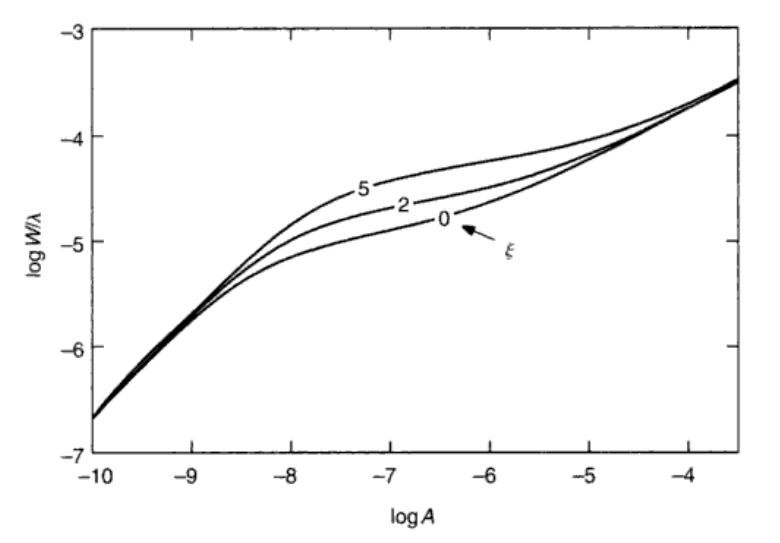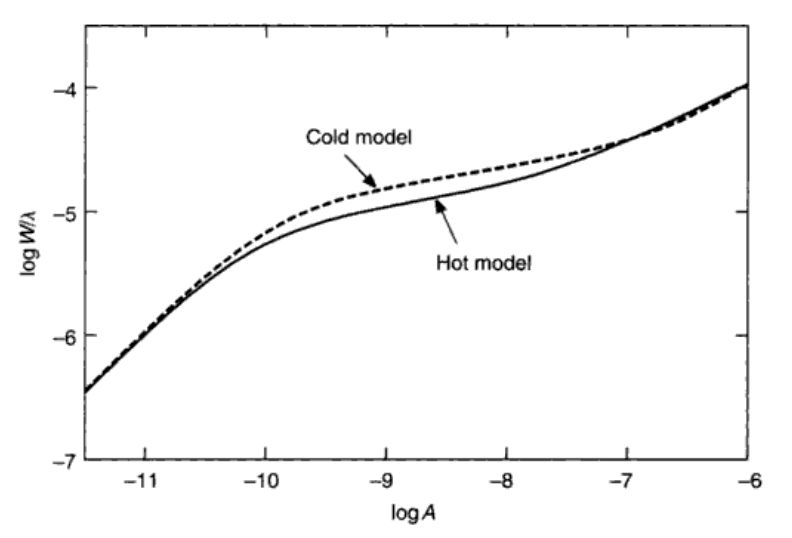### 金属丰度的确定

$$\frac{w}{\lambda}$$确定之后，$$(16.4)$$右边可以有无数个解。那么我们取两条线（1和2），硬是代入这个$$\frac{w}{\lambda}$$里面，就有

\begin{align} \log{\frac{w}{\lambda}} &= \log{C} + \log{A_1} + \log{g_{n1}f_1\lambda_1} - \theta_{ex} \chi_1 - \log{\kappa_{\nu 1}} \\ &= \log{C} + \log{A_2} + \log{g_{n2}f_1\lambda_2} - \theta_{ex} \chi_2 - \log{\kappa_{\nu 2}} \end{align} $\Rightarrow \Delta \log{A} = \log{\frac{g_{n1}f_1\lambda_1}{g_{n2}f_2\lambda_2}} - \log{\frac{\kappa_{\nu 1}}{\kappa_{\nu 2}}} - \theta_{ex} (\chi_1 - \chi_2) \tag{16.5}$

## 较差测量

$\log{\frac{A}{A_\mathrm{ref}}} = \log{\frac{w}{w_\mathrm{ref}}} - \log{\frac{\kappa_{\nu}}{\kappa_{\nu\mathrm{ref}}}} - (\theta_{ex}-\theta_{ex\mathrm{ref}}) \chi \tag{16.7}$

## 丰度指标

UBV测光可以在一定程度上反应金属丰度，因为三个波段上的谱线数量依次递减，而金属丰度越高，自然吸收越多。所以恒星在双色图$$(U-B)$$-$$(B-V)$$上的位置可以反映它的金属丰度。当然得先改掉红化。

## 太阳丰度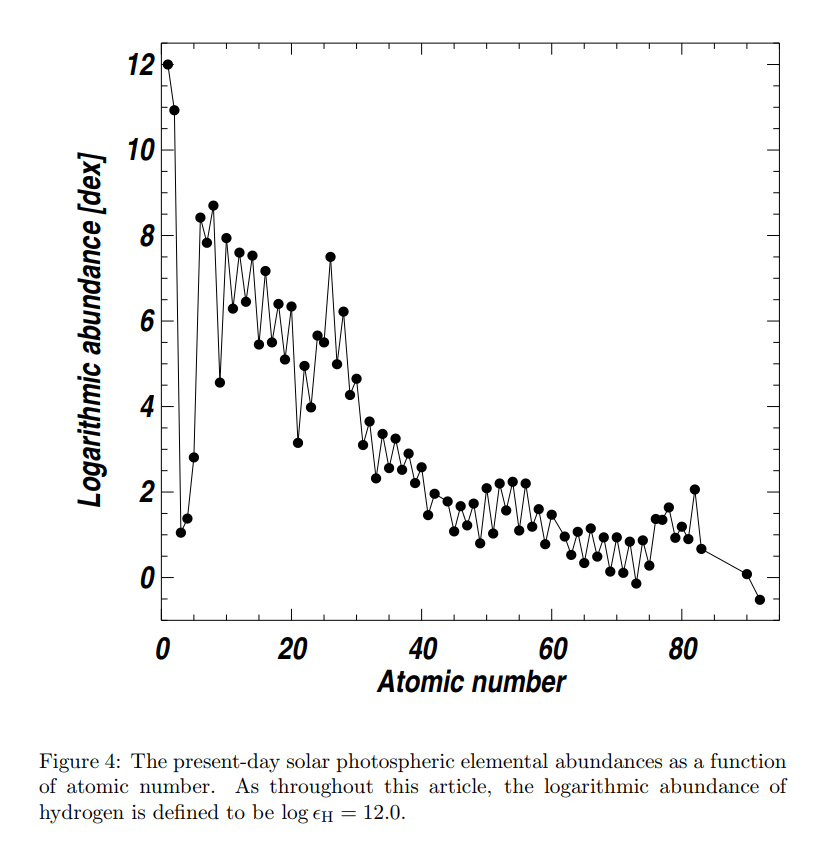Asplund+2009

## 银河系的恒星丰度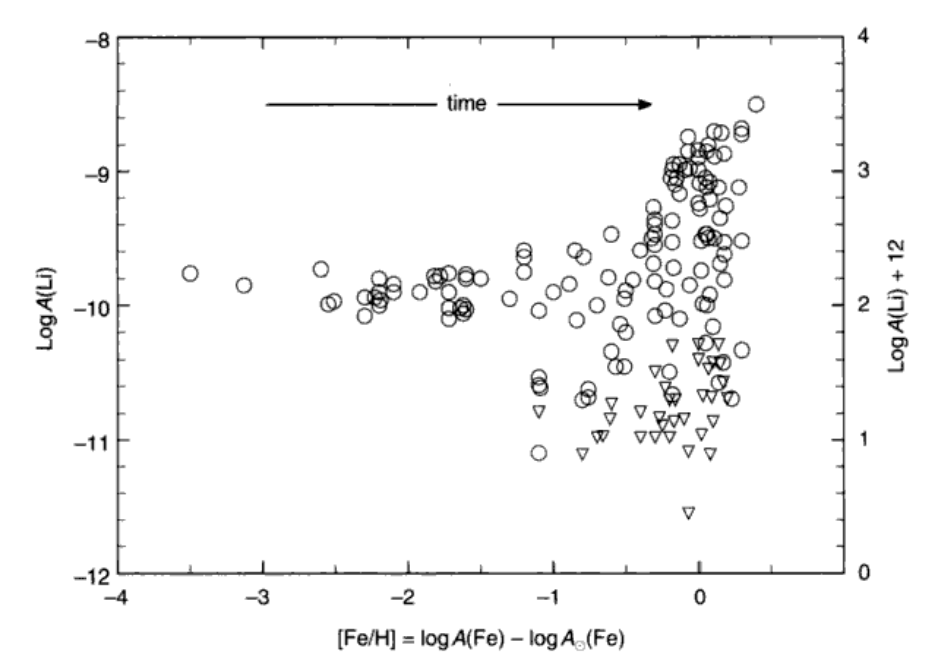### 锂丰度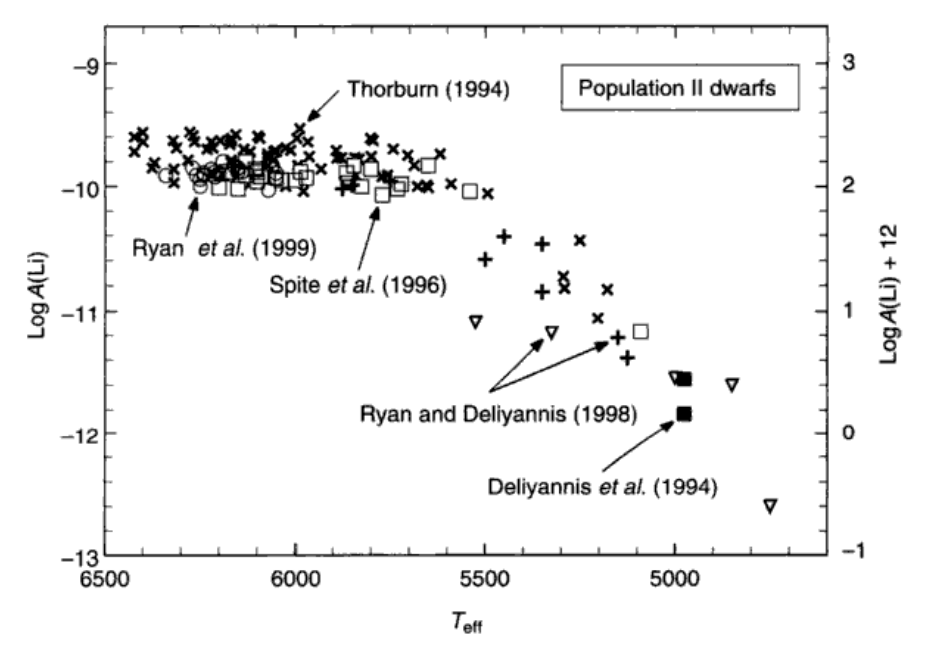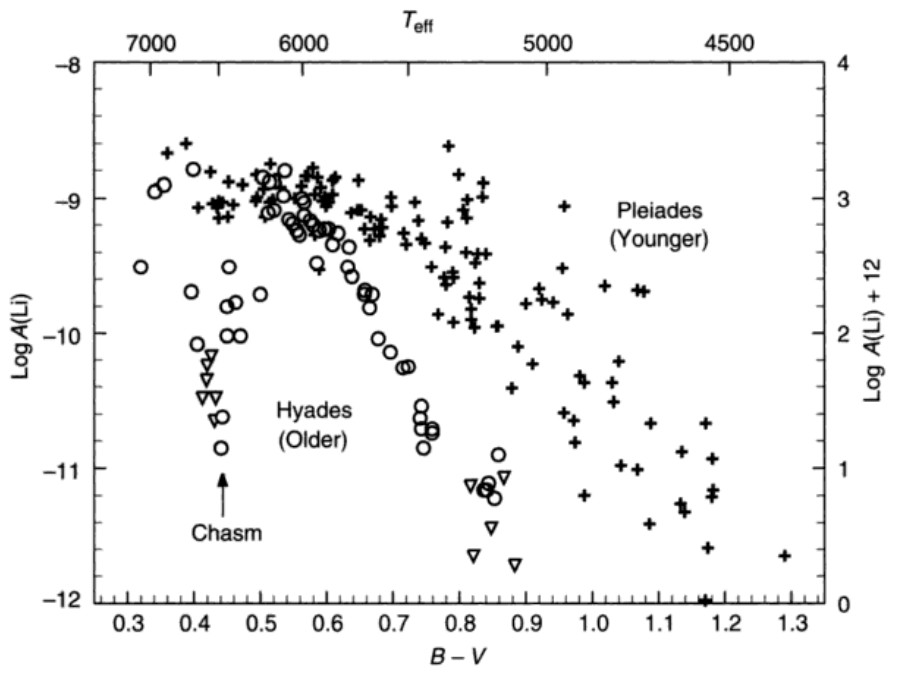Be也可以用来探测恒星内部结构。

### 碳丰度

CNO循环的事情。又和韩国人联系上了。碳12和碳13的比值也会随着恒星演化发生改变，从最初的90跌到15左右。

## 碎碎念